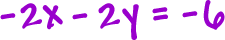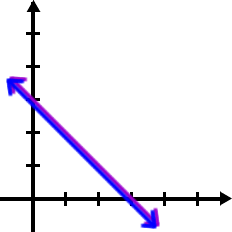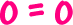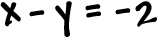The other freaky thing that can happen is that the two lines can be lying right on top of each other -- they are the same line.

Let's graph these two lines:So, what the heck does this mean for our answer?  Every single
(xy) point that appears in one line appears in the other.  So, everyx and y combination that works in one equation, works in the other equation.  And how many points are in a line?  That's right, baby, there's an infinite number of them!  So how many points that work in one line work in the other?  An infinite number.

 These are the same line, so there are an infinite number of solutions.

How is this little critter going to announce itself to you?

When you're solving by elimination or substitution...  you'll be going along...  and you'll get this:A true statement, but not very helpful unless you know what you're doing -- and you do!

TRY IT:

Solve by either elimination or substitution.  Then, check your answer by graphing: